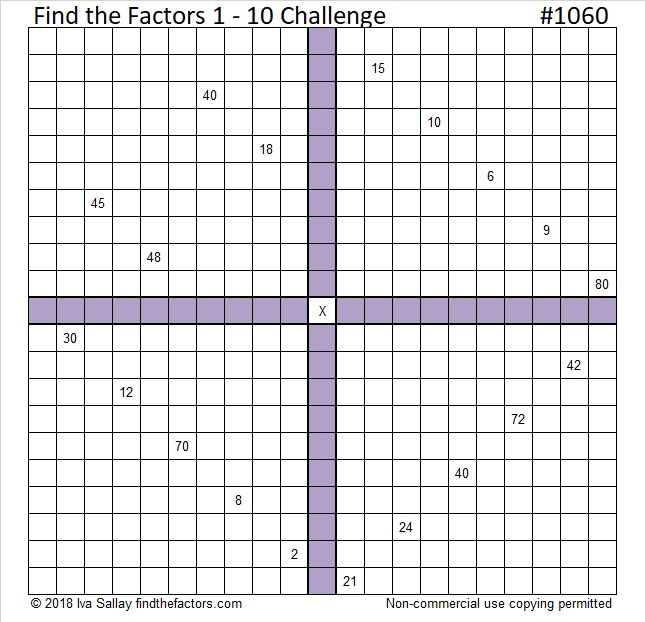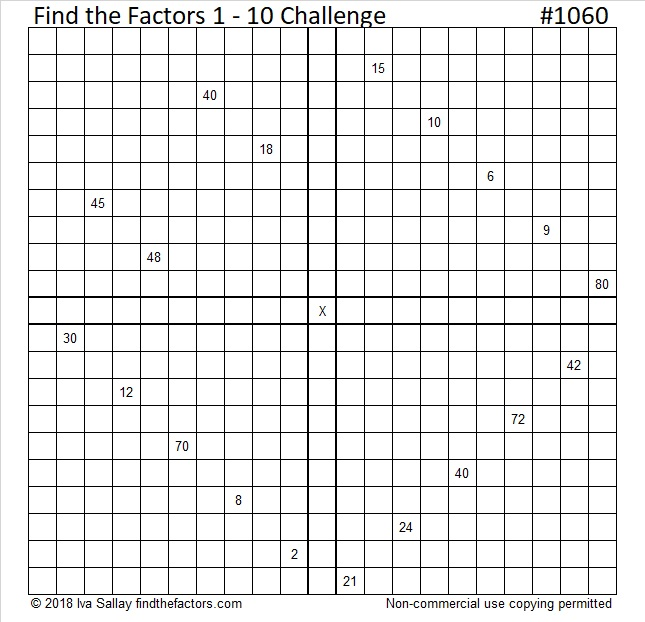# 1060 A Challenge for Justin’s Birthday

Justin had no problems solving the puzzle I made for his last birthday, so this year I’ve made it tougher. Write the numbers from 1 to 10 in each of the four purple sections of the puzzle so that the clues are the products of the corresponding factors. Like always, there is only one solution. This puzzle can still be solved entirely by using logic and knowledge of basic multiplication and division facts. Will Justin be able to figure it out? I’m anxious to find out. Happy birthday, Justin!Here’s the same puzzle without added color if that’s better for printing.Print the puzzles or type the solution in this excel file: 10-factors-1054-1062

Now here are some things I’ve learned about the number 1060:

• 1060 is a composite number.
• Prime factorization: 1060 = 2 × 2 × 5 × 53, which can be written 1060 = 2² × 5 × 53
• The exponents in the prime factorization are 2, 1, and 1. Adding one to each and multiplying we get (2 + 1)(1 + 1)(1 + 1) = 3 × 2 × 2 = 12. Therefore 1060 has exactly 12 factors.
• Factors of 1060: 1, 2, 4, 5, 10, 20, 53, 106, 212, 265, 530, 1060
• Factor pairs: 1060 = 1 × 1060, 2 × 530, 4 × 265, 5 × 212, 10 × 106, or 20 × 53,
• Taking the factor pair with the largest square number factor, we get √1060 = (√4)(√265) = 2√265 ≈ 32.557641060 is the sum of consecutive prime numbers three different ways:
It is the sum of the prime numbers from 2 to 97, that’s all the prime numbers less than 100.
83 + 89 + 97 + 101 + 103 + 107 + 109 + 113 + 127 + 131 = 1060; that’s 10 consecutive primes
257 + 263 + 269 + 277 = 1060; that’s 4 consecutive primes

32² + 6² = 1060
24² + 22² = 1060

1060 is the hypotenuse of FOUR Pythagorean triples:
92-1056-1060
560-900-1060
636-848-1060
384-988-1060

1060 is a palindrome in some other bases:
884 in BASE 11
424 in BASE 16
202 in BASE 23

This site uses Akismet to reduce spam. Learn how your comment data is processed.